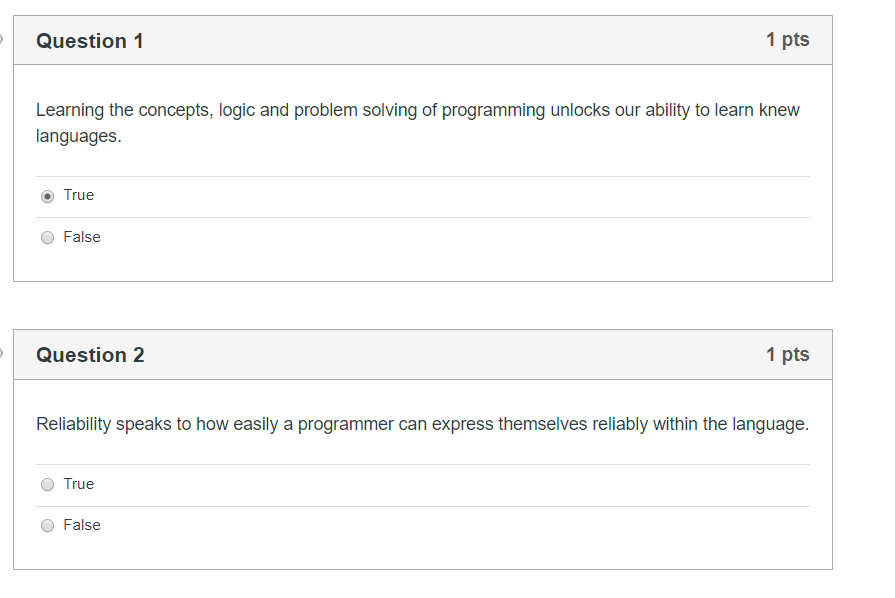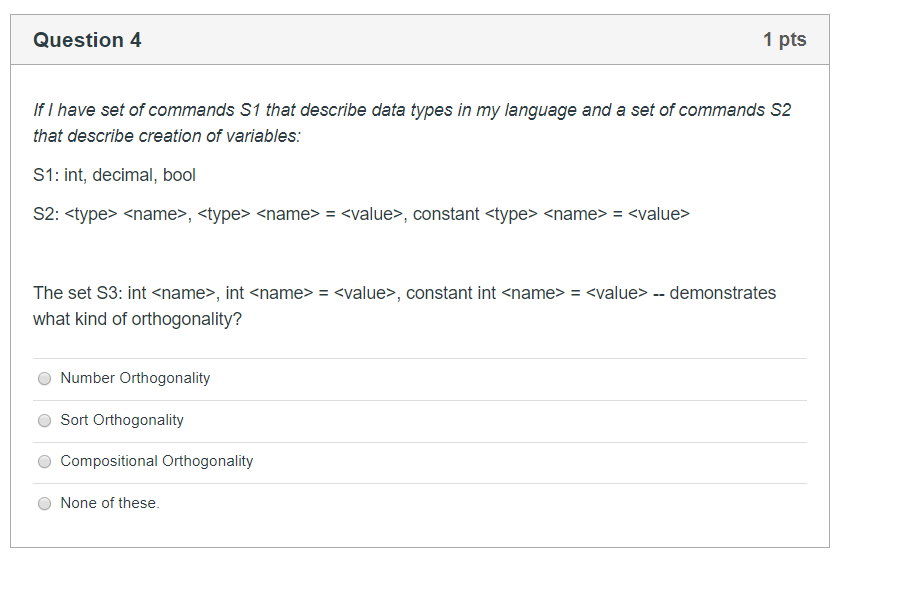# Question i need the answers to the following questions. if the image is too small right click and open in a new tab for an enlarged view. Question 1 1 pts Learning the concepts, logic and problem solving of programming unlocks our ability to learn knew languages. True False Question 2 1 pts Reliability speaks to how easily a programmer can express themselves reliably within the language. True False Question 4 1 pts If I have set of commands S1 that describe data types in my language and a set of commands S2 that describe creation of variables: S1: int, decimal, bool S2: <type> <name>, <type> <name> - <value>, constant <type> <name> = <value> The set S3: int <name>, int <name> = <value>, constant int <name> = <value> -- demonstrates what kind of orthogonality? Number Orthogonality Sort Orthogonality Compositional Orthogonality None of these05RZKO The Asker · Computer Sciencei need the answers to the following questions. if the image is too small right click and open in a new tab for an enlarged view.

Transcribed Image Text: Question 1 1 pts Learning the concepts, logic and problem solving of programming unlocks our ability to learn knew languages. True False Question 2 1 pts Reliability speaks to how easily a programmer can express themselves reliably within the language. True False Question 4 1 pts If I have set of commands S1 that describe data types in my language and a set of commands S2 that describe creation of variables: S1: int, decimal, bool S2: , - , constant = The set S3: int , int = , constant int = -- demonstrates what kind of orthogonality? Number Orthogonality Sort Orthogonality Compositional Orthogonality None of these
More
Transcribed Image Text: Question 1 1 pts Learning the concepts, logic and problem solving of programming unlocks our ability to learn knew languages. True False Question 2 1 pts Reliability speaks to how easily a programmer can express themselves reliably within the language. True False Question 4 1 pts If I have set of commands S1 that describe data types in my language and a set of commands S2 that describe creation of variables: S1: int, decimal, bool S2: , - , constant = The set S3: int , int = , constant int = -- demonstrates what kind of orthogonality? Number Orthogonality Sort Orthogonality Compositional Orthogonality None of these